Type
Sale/Rent
Min Price
Max Price
Min Beds
Max Beds

# Property For Rent Showing properties 1 to 12 of 26

Sort by
• Let agreedLet agreed £575 / month
• Let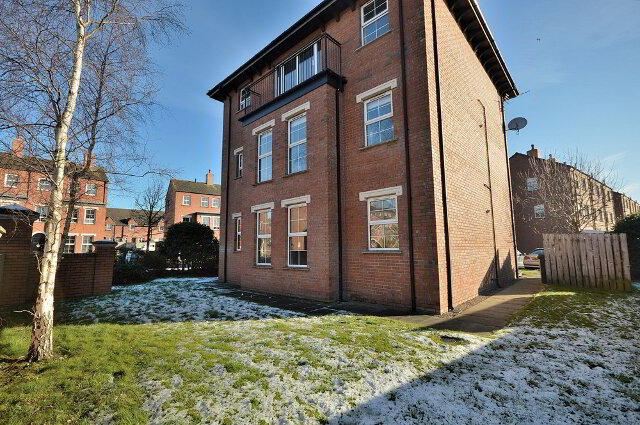LET
• Let agreedLet agreed £575 / month
• LetLET
• Let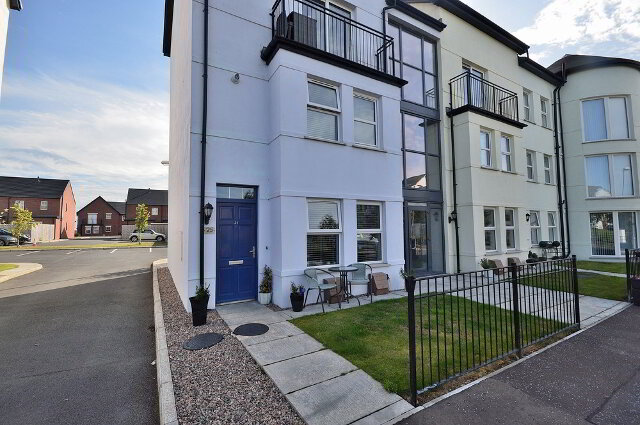LET
• Let agreed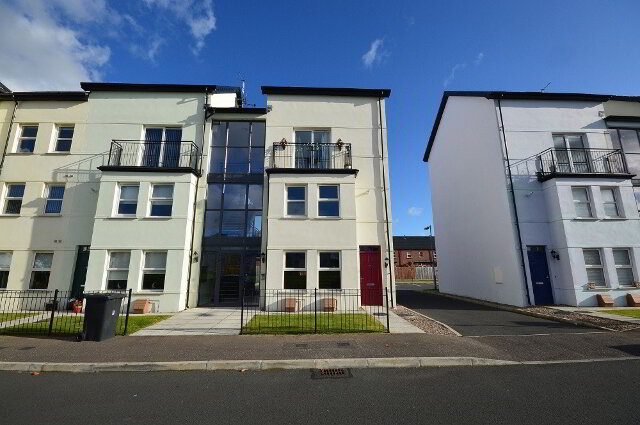Let agreed £550 / month
• Let agreed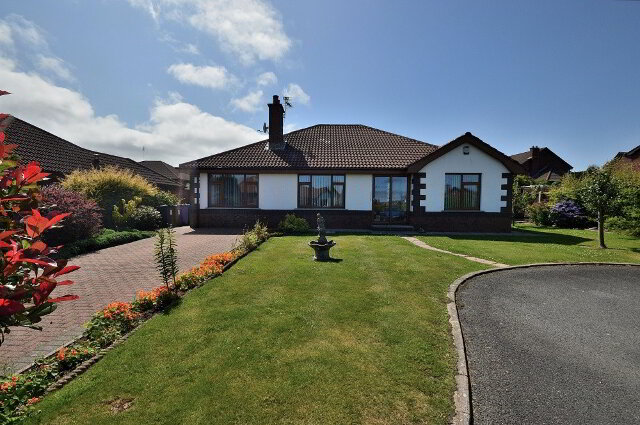Let agreed £900 / month
• Let agreed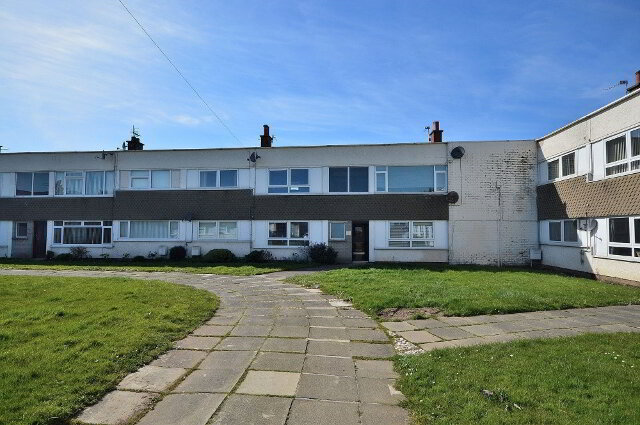Let agreed £475 / month
• Let agreed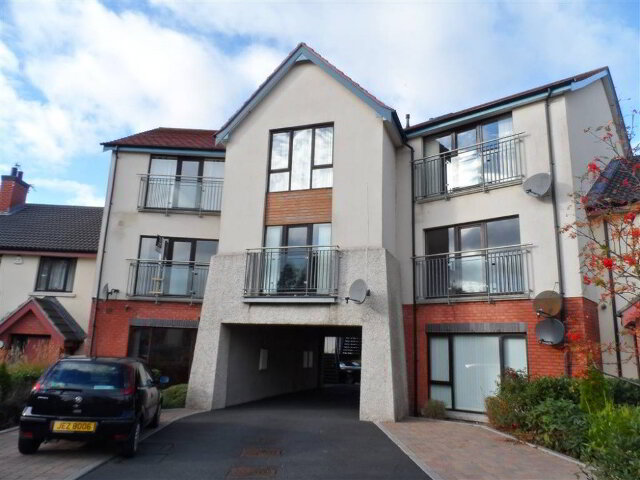Let agreed £500 / month
• Let agreed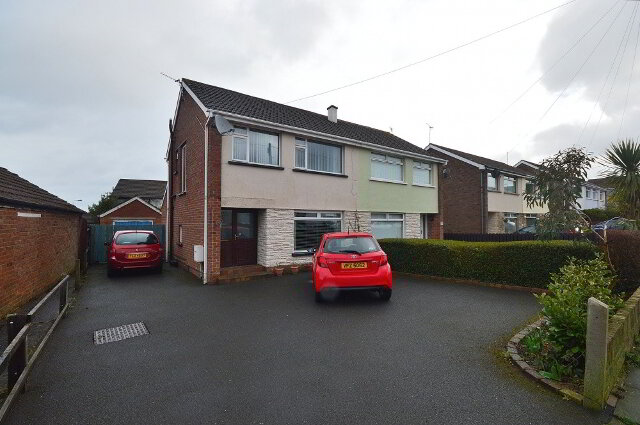Let agreed £600 / month
• Let agreed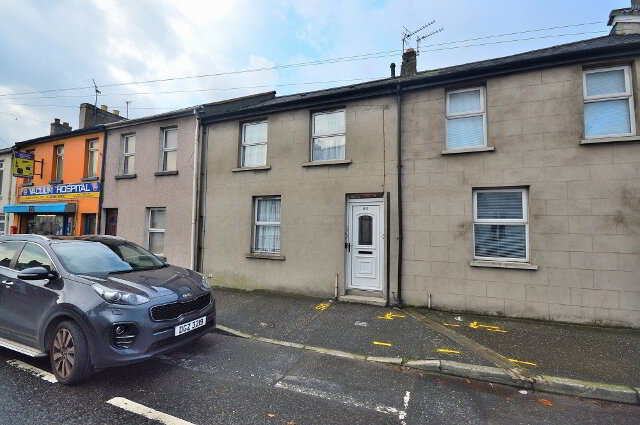Let agreed £450 / month
• Let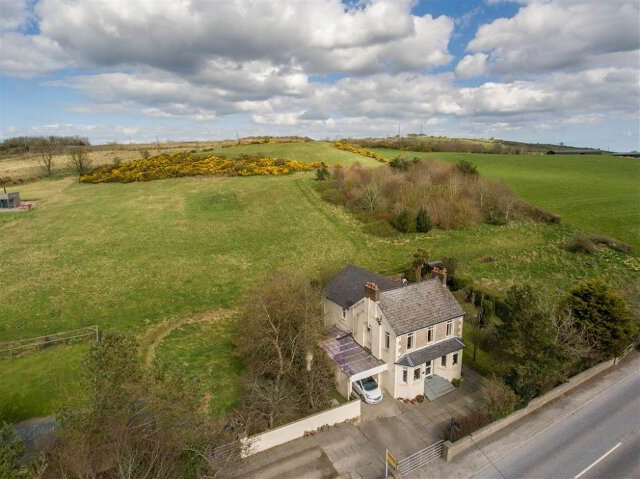LET